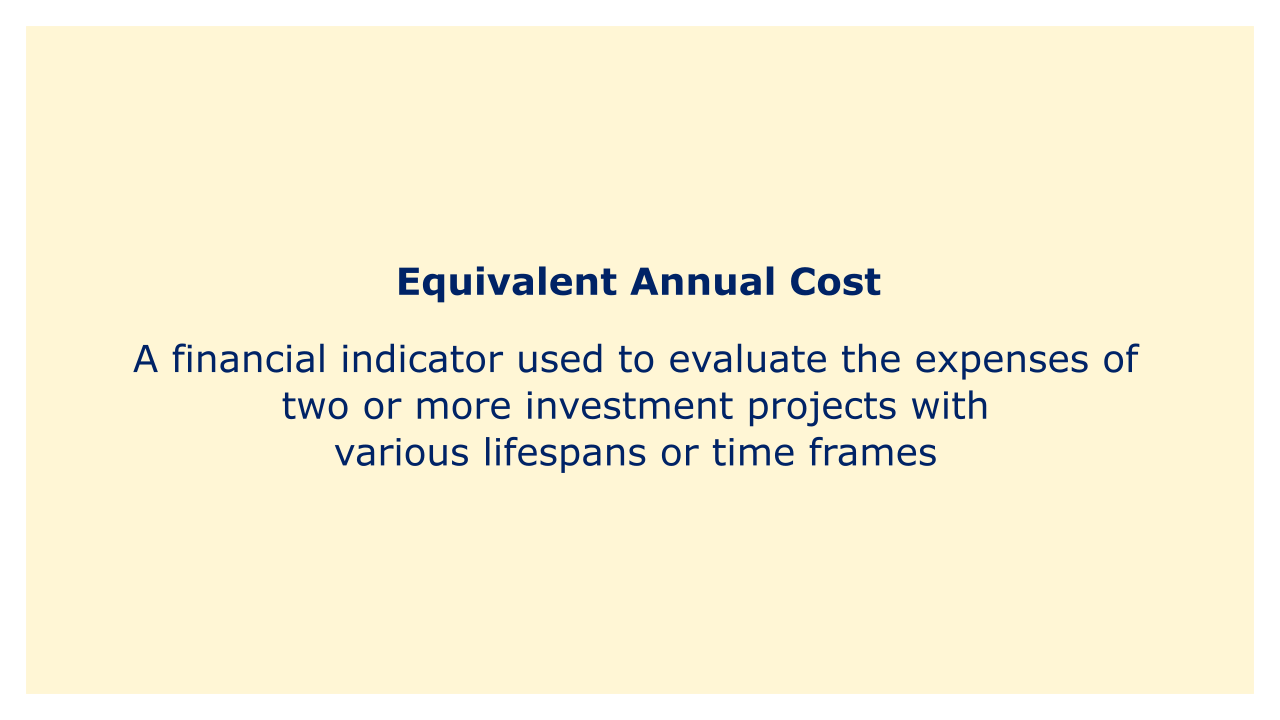# Equivalent Annual CostImage: Moneybestpal.com

### The term "equivalent annual cost" (EAC) refers to a financial indicator used to evaluate the expenses of two or more investment projects with various lifespans or time frames. As a single equal annual payment over the course of an investment's life, EAC shows the annual cost of the investment.

It is computed by considering the present value of all costs related to an investment, such as the upfront, ongoing, and maintenance expenditures, and distributing them over the course of the investment using a discount rate.

The EAC formula is as follows:

EAC = (A / PVIFA) + (S / PVIF)

where A represents the investment's annualized cost, S is its salvage value, PVIFA is its present value interest factor for annuities, and PVIF is its present value interest factor for a single payment.

The whole cost of the investment, including any upfront fees, ongoing costs, maintenance costs, and salvage value, must first be ascertained in order to compute EAC. The next step is to use a discount rate that takes into account both the investment's risk and the time value of money to reduce each of these cash flows to their present value. All cash flows are then distributed evenly across the investment's life to determine the comparable yearly cost after they have been converted to present value terms for all of them.

Since it enables an apples-to-apples comparison of the annual expenses of each investment, the EAC statistic is helpful for evaluating the costs of investments with various lifetimes. Investors and analysts can compare EAC against several investment possibilities
Tags